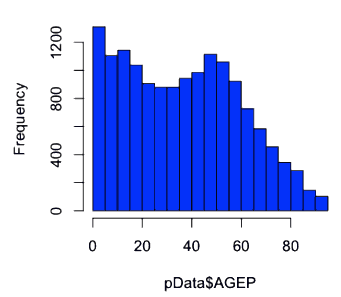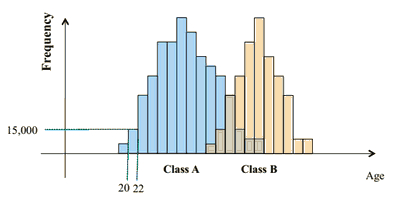# ML Wiki

## Histogram

Histogram is a graphical representation of the Distribution of data

• Bins: the intervals used in a histogram. The data must be separated into mutually exclusive and exhaustive bins
• Cutpoints: the values that define the beginning and the end of the bins
• Frequency: the count of the number of the data values in each bin
• The peaks in the distribution are called modes
• so the variables you plot must be Quantitative Variables

hist(d$age, col="blue") • Params • breaks=100 - how many bars in the histogram •• here we have 19 bins, and two modes ## Bivariate Analysis It can also be useful for Exploratory Data Analysis of two variables Consider this example • we have two classes of customers:$A$and$B$• and we want to build a model that can distinguish them • so we can create a histogram that shows the distribution of age w.r.t. to class attribute •• can see that age and class are not independent: there is strong correlation between them: • if age is lower then some value (say 30), all belong to class$A$• if greater than other value - all always belong to class$B\$
• can learn that just using a simple histogram

## Cumulative Histogram

Usual histogram estimates the Probability Density Function